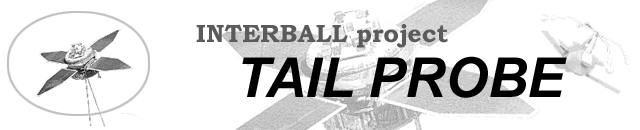Introduction and presentation of two coordinate systems used in attitude motion model

How to get matrix of transformaton using attitude coefficients data

Attitude coefficients data files

Software and example

Data availibility information presented by diagrams

Angles between the angular momentum vector (~the spin axis) and the Sun direction